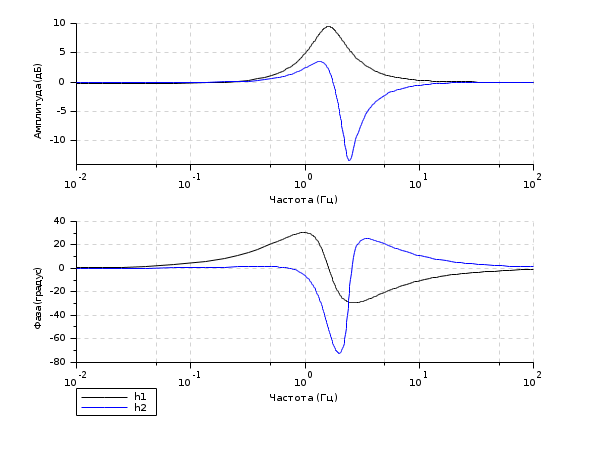Change language to:
English - Français - 日本語 - Português -

See the recommended documentation of this function

Scilab help >> CACSD > Plot and display > bode

# bode

Bode plot

### Calling Sequence

```bode(sl,[fmin,fmax] [,step] [,comments] )

### Arguments

sl

`syslin` list (SISO or SIMO linear system) in continuous or discrete time.

fmin,fmax

real (frequency bounds (in Hz))

step

real (logarithmic step.)

vector of character strings (captions).

frq

row vector or matrix (frequencies (in Hz) ) (one row for each SISO subsystem).

db

row vector or matrix ( magnitudes (in Db)). (one row for each SISO subsystem).

phi

row vector or matrix ( phases (in degree)) (one row for each SISO subsystem).

repf

row vector or matrix of complex numbers (complex frequency response).

### Description

Bode plot, i.e magnitude and phase of the frequency response of `sl`.

`sl` can be a continuous-time or discrete-time SIMO system (see `syslin`). In case of multi-output the outputs are plotted with different symbols.

The frequencies are given by the bounds `fmin,fmax` (in Hz) or by a row-vector (or a matrix for multi-output) `frq`.

`step` is the ( logarithmic ) discretization step. (see `calfrq` for the choice of default value).

`comments` is a vector of character strings (captions).

`db,phi` are the matrices of modulus (in Db) and phases (in degrees). (One row for each response).

`repf` matrix of complex numbers. One row for each response.

Default values for `fmin` and `fmax` are `1.d-3`, `1.d+3` if `sl` is continuous-time or `1.d-3`, `0.5`/sl.dt (nyquist frequency) if `sl` is discrete-time. Automatic discretization of frequencies is made by `calfrq`.

The

• datatips
• tool may be used to display data along the phase and modulus curves.

### Examples

```s=poly(0,'s')
h=syslin('c',(s^2+2*0.9*10*s+100)/(s^2+2*0.3*10.1*s+102.01))
clf();bode(h,0.01,100);``````s=poly(0,'s')
h1=syslin('c',(s^2+2*0.9*10*s+100)/(s^2+2*0.3*10.1*s+102.01))
num=22801+4406.18*s+382.37*s^2+21.02*s^3+s^4;
den=22952.25+4117.77*s+490.63*s^2+33.06*s^3+s^4
h2=syslin('c',num/den);

clf();bode([h1;h2],0.01,100,['h1';'h2'])```